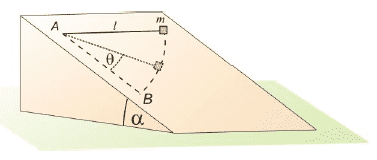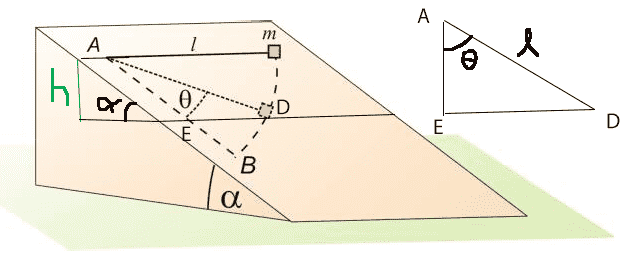# Finding the speed's dependence on angle θ

• Rain10399
In summary, the problem at hand is to find the relationship between the speed of an object and the angle of an inclined plane, assuming an ideal string and no friction. The suggested approach is to use the conservation of energy, but the challenge lies in expressing the relevant height in terms of the angle. The use of a coordinate system and the Lagrangian formulation of mechanics may be helpful in solving this problem.

## Homework Statement

Find v(θ), the dependence of the speed of the object on the angle θ. The string is ideal and there's no friction.-

## The Attempt at a Solution

I'm quite sure I should use the conservation of energy mgh = mv2/2, but I don't know how to express h with the help of the angle θ

#### Attachments

Can you express position on the surface of the inclined plane in terms of the angle? Lay down a coordinate system on the surface of the plane if it helps. I would suggest anchoring that coordinate system, putting its origin at point A.

Edit: Your approach of using conservation of energy will work. As you say, the problem is figuring out height as a function of angle.

Last edited:
•Rain10399
Rain10399 said:

## Homework Statement

Find v(θ), the dependence of the speed of the object on the angle θ. The string is ideal and there's no friction.

View attachment 230881

-

## The Attempt at a Solution

I'm quite sure I should use the conservation of energy mgh = mv2/2, but I don't know how to express h with the help of the angle θ

Have you taken the "Lagrangian" formulation of mechanics yet? If so, the problem will be straightforward; if not, you will have a bit more work to do, resolving the force of gravity into the appropriate components related to the plane, etc.

•Rain10399
jbriggs444 said:
Can you express position on the surface of the inclined plane in terms of the angle? Lay down a coordinate system on the surface of the plane if it helps. I would suggest anchoring that coordinate system, putting its origin at point A.

Edit: Your approach of using conservation of energy will work. As you say, the problem is figuring out height as a function of angle.

I laid down the coordinate system on the surface of the plan at the origin A, but I didn't manage to express the first position of m in terms of the angle θ. I drew a straight line between the first position of m and B, but the triangle with the angle θ in it is not right, so I'm not sure how to proceed from there.

Ray Vickson said:
Have you taken the "Lagrangian" formulation of mechanics yet? If so, the problem will be straightforward; if not, you will have a bit more work to do, resolving the force of gravity into the appropriate components related to the plane, etc.

I haven't taken the Lagrangian formulation of mechanics yet (I'm in high school and I'm not sure if it's in our curriculum), but I watched Michel van Biezen's first video on Lagrangian Mechanics on Youtube.

Rain10399 said:
I laid down the coordinate system on the surface of the plan at the origin A, but I didn't manage to express the first position of m in terms of the angle θ.
The starting position is "l" over and zero down.

Rain10399 said:
View attachment 230896
So I found h = sin α * AC, and now I'm trying to find AC in terms of angle θ.
I thought mass m was on a string. Why is AC relevant?

•Rain10399
I'm trying to find the height at which is m in terms of angle θ, so that I can replace h with the angle in formula mgh = mv2/2. I don't know how else to solve the problem.

Rain10399 said:
I'm trying to find the height at which is m in terms of angle θ, so that I can replace h with the angle in formula mgh = mv2/2. I don't know how else to solve the problem.
The relevant height h is above (or below) what baseline?

The relevant height is from the base of the plane to the position of A or to m's initial position (which have the same height)

Rain10399 said:
The relevant height is from the base of the plane to the position of A or to m's initial position (which have the same height)
That is incorrect.

You should be working on a conservation of energy argument. You know the kinetic energy at the starting point. You know the change in potential energy between starting point and ending point.

Whether the entire plane is on a table, on the floor or on the roof of a building is irrelevant.

•Rain10399
jbriggs444 said:
That is incorrect.

You should be working on a conservation of energy argument. You know the kinetic energy at the starting point. You know the change in potential energy between starting point and ending point.

Whether the entire plane is on a table, on the floor or on the roof of a building is irrelevant.

Oh! Then what is the relevant height?
At the start there's no kinetic energy, only potential energy, so I still need to express the h in mgh.

Rain10399 said:
Oh! Then what is the relevant height?
At the start there's no kinetic energy, only potential energy, so I still need to express the h in mgh.
You want to know how much potential energy has changed between the starting point and the ending point. The height difference between starting point and ending point would be of some interest.

•Rain10399
jbriggs444 said:
You want to know how much potential energy has changed between the starting point and the ending point. The height difference between starting point and ending point would be of some interest.

So can h be the difference between A and D (or E. D and E are supposed to be at the same height, but I'm not sure if that can be seen on the drawing)? Then h = sin α * AE and AE = cos θ * l.
So mg * sin α * cos θ * l = mv2/2, and from there I can express v in terms of θ.However, I had to make he assumption that AED is a right triangle in order to express AE in terms of θ.

#### Attachments

•jbriggs444
Rain10399 said:
However, I had to make he assumption that AED is a right triangle in order to express AE in terms of θ.
Seems a reasonable assumption. You are measuring the angle from a reference that we assume is directly downslope. The horizontal lines across the slope will be at right angles to this.

•Rain10399
jbriggs444 said:
Seems a reasonable assumption. You are measuring the angle from a reference that we assume is directly downslope. The horizontal lines across the slope will be at right angles to this.

Oh, yes, you're right.
Thank you for helping me with this problem!